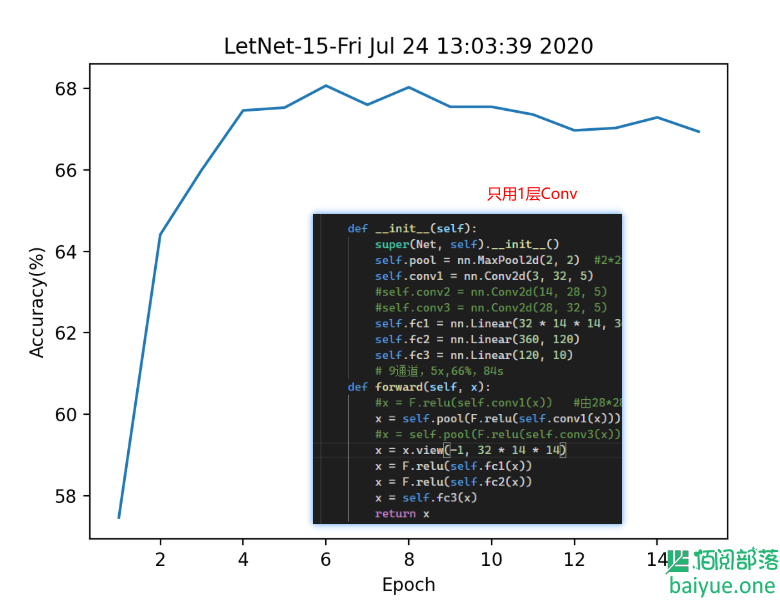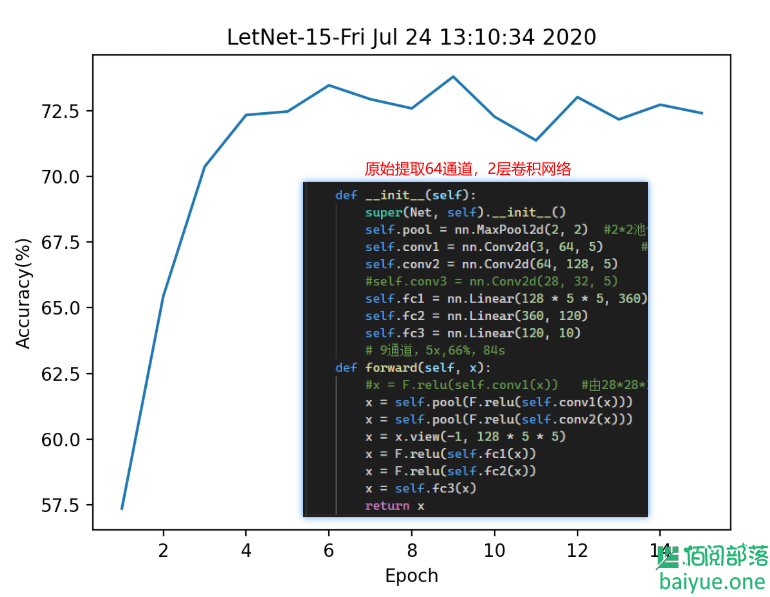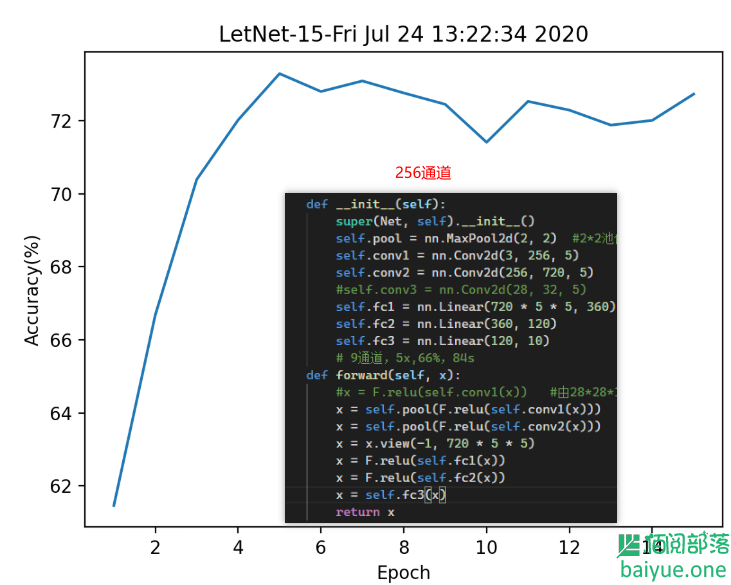• 欢迎访问佰阅部落
• 如果您觉得本站非常有看点，那么赶紧使用Ctrl+D 收藏吧

# 图像分类训练-使用LetNet卷积网络分类CIFAR10

2年前 (2020-07-24) 1764次浏览## 0. 图像分类训练一般步骤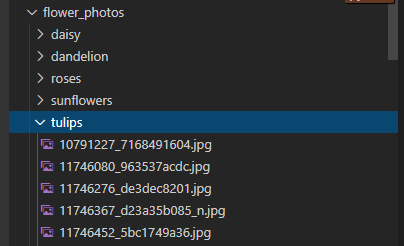`CIFAR10`是比较经典的数据集，包含5万张训练图像和1万张测试图像，大小均为32*32像素。

## 1. LetNet卷积神经网络

LetNet网络可以用下图说明：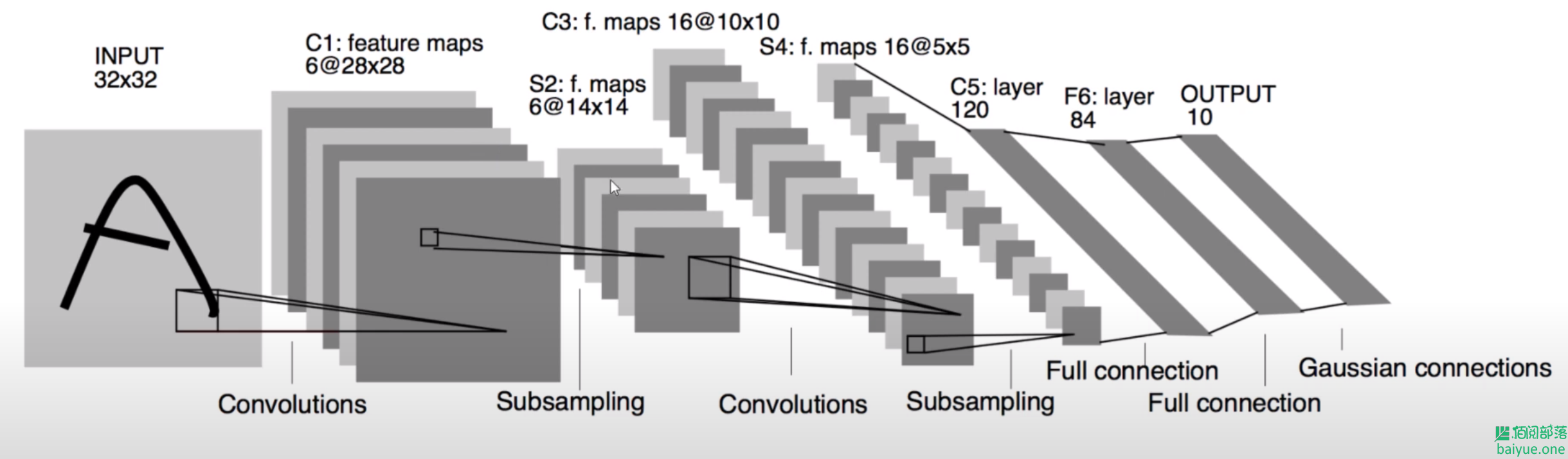``````class Net(nn.Module):
def __init__(self):
super(Net, self).__init__()
self.conv1 = nn.Conv2d(3, 6, 5)
self.pool = nn.MaxPool2d(2, 2)
self.conv2 = nn.Conv2d(6, 16, 5)
self.fc1 = nn.Linear(16 * 5 * 5, 120)
self.fc2 = nn.Linear(120, 84)
self.fc3 = nn.Linear(84, 10)

def forward(self, x):
x = self.pool(F.relu(self.conv1(x)))
x = self.pool(F.relu(self.conv2(x)))
x = x.view(-1, 16 * 5 * 5)
x = F.relu(self.fc1(x))
x = F.relu(self.fc2(x))
x = self.fc3(x)
return x
``````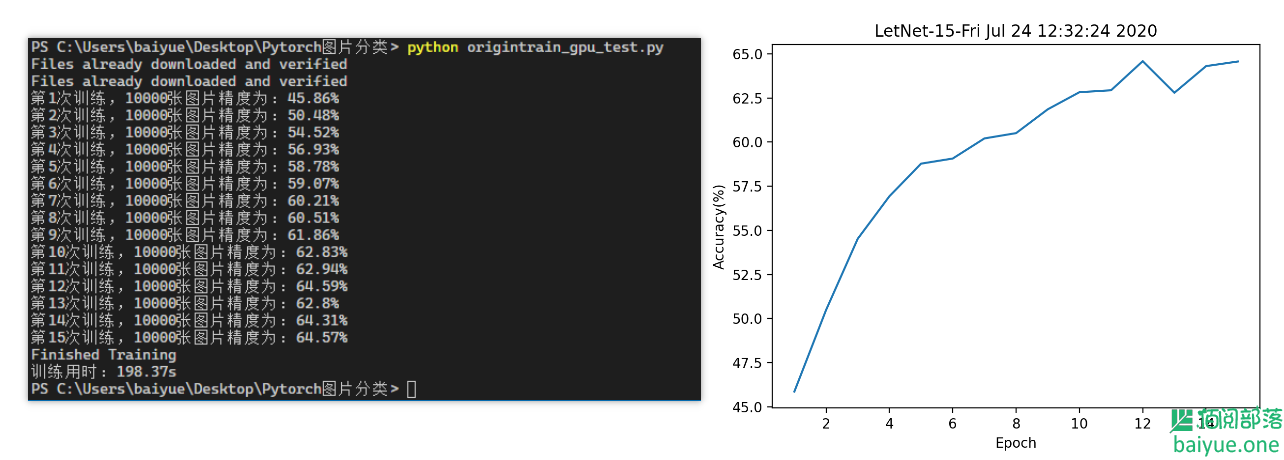64%的精度对我们而言比较牵强，因此我又开始调整了下这个神经网络，扩展conv层为3层。

``````class Net(nn.Module):
def __init__(self):
super(Net, self).__init__()
self.pool = nn.MaxPool2d(2, 2)  #2*2池化层，步长为2
self.conv1 = nn.Conv2d(3, 14, 5)     #
self.conv2 = nn.Conv2d(14, 28, 5)    #
self.conv3 = nn.Conv2d(28, 32, 5)    #增加一层
self.fc1 = nn.Linear(32 * 4 * 4, 360)
self.fc2 = nn.Linear(360, 120)
self.fc3 = nn.Linear(120, 10)
# 9通道，5x,66%，84s
def forward(self, x):
x = F.relu(self.conv1(x))   #32*32*3cov>>28*28*14
x = self.pool(F.relu(self.conv2(x)))    #28*28*14>>24*24*28>>12*12*28
x = self.pool(F.relu(self.conv3(x)))    #12*12*28>>8*8*32>>4*4*32
x = x.view(-1, 32 * 4 * 4)
x = F.relu(self.fc1(x))
x = F.relu(self.fc2(x))
x = self.fc3(x)
return x
``````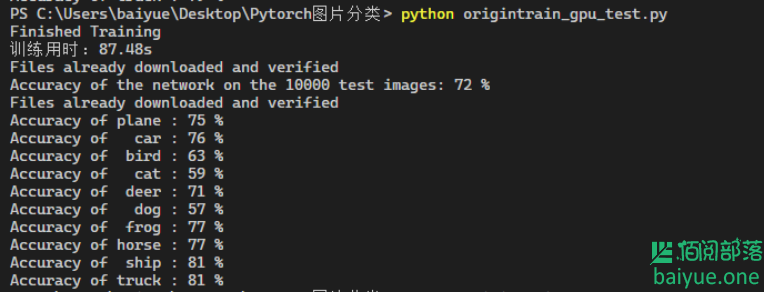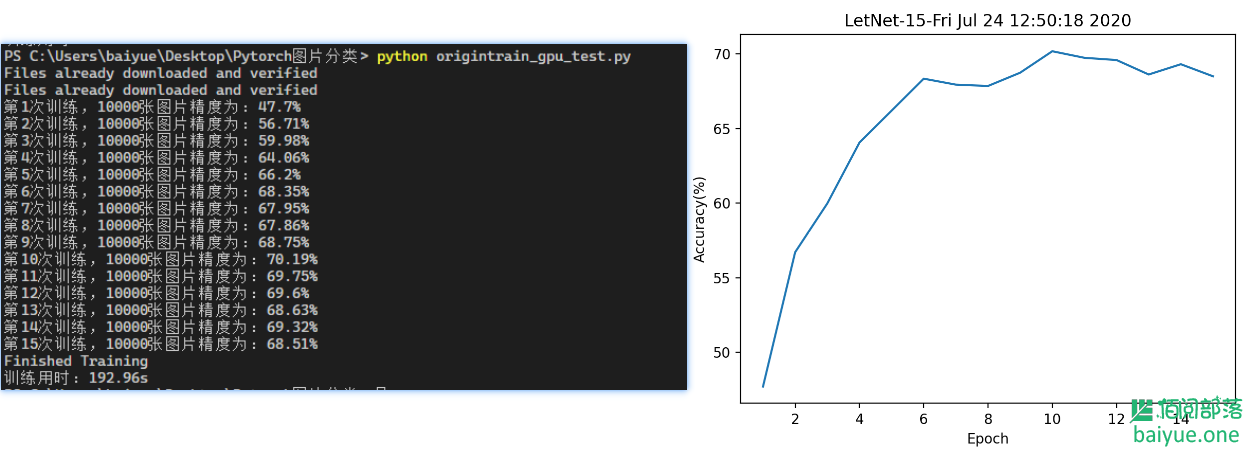## 2. 讨论